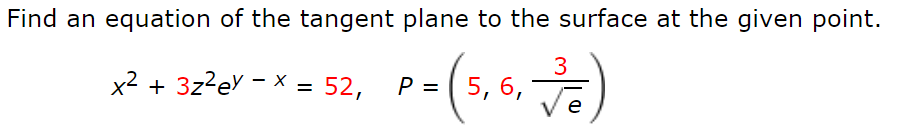# Find an equation of the tangent plane to the surface at the given point. 3 x2 + 3z?ev - x = 52, P = ( 5, 6, %3D e

Question

Find an equation of the tangent plane to the surface at the given point.

x2 + 3z2ey − x = 52,    P = (5,6,3/sqrt(e))help_outlineImage TranscriptioncloseFind an equation of the tangent plane to the surface at the given point. 3 x2 + 3z?ev - x = 52, P = ( 5, 6, %3D e fullscreen

### Want to see this answer and more?

Experts are waiting 24/7 to provide step-by-step solutions in as fast as 30 minutes!*

*Response times may vary by subject and question complexity. Median response time is 34 minutes for paid subscribers and may be longer for promotional offers.
Tagged in
Math
Calculus

### Other